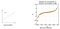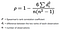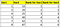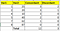# Day 39 — Spearman and Kendall’s rank for monotonic relationships

Previously, we have seen some correlation techniques to measure the independency between the input features(Pearson, VIF, ANOVA & Chi-Squared tests). In this article, let’s explore some more methods. The only objective for these approaches is to identify how strongly the input and output target is correlated. As well as, to detect if the independent features are related to one another(multicollinearity).

Table of contents:

• Spearman Correlation(num Vs num)
• Kendall’s rank Correlation(num Vs num)

Spearman Correlation:- To find the relationship between two continuous variables we can incorporate this procedure. One may wonder, we already have the Pearson Correlation to do the same. Then, how Spearman is different from Pearson?. In the case of the Pearson correlation, the relation should be linear, whereas, Spearman shows the monotonic link between two features.Fig 1 — showed linear and Monotonic Relationship took from Wikipedia

From the above picture, we can say if two variables are linearly related, then the rate of change in both variables are the same in a similar direction. While a monotonic relation depicts the change in one variable follows the same direction as that of change in another variable even though the rate of change could be different.

How to compute the correlation mathematically? Since the aim is to validate the monotonic relationship between the variables, the correlation is assessed based on the ordering of the data. It comes under the category of the non-parametric test(no assumption about the variable is made). First, we need to order the two variables and give ranks to feature values. This is followed by applying the Spearman correlation formula.The formula for Spearman Correlation

Here ‘d’ represents the difference between the two ranks of each observation.Sample data for Spearman correlation

In the example data, we have two variables ‘Var1’ & ‘Var2’ and the respective ordering is populated under ‘Rank for Var1’ & ‘Rank for Var2’. The summation of the difference between the two ranks is zero as we have complete matching order. The final value of the correlation coefficient is 1 (i.e) implying there is a strong monotonic relationship between the variables. Notes: The maximum value for the correlation is +1 and the minimum value is -1.

Kendall’s rank correlation: Another type of correlation procedure which is non-parametric. Kendall is preferred over the Spearman when the sample size is small because of its robustness. It works on the principle of Concordant and discordant. Let’s take an example to understand the concept better.Example for Kendall’s rank correlation

Var1(anyone variable) is ordered in the ascending order, the Concordant values are calculated based on the number of values higher than the current value in the Var2. So if we take first value 25, then there are totally 3 records which are greater than 25. But if we take 40, then the value will be 2. The same concept applies to the discordant, but the values should be less than the current one. So for the first record, we have 2 records with smaller values than the present one.

Kendall’s corr coeff = (C — D) / (C + D) C — Concordant, D — Discordant

Kendall’s corr = 12–3 / 12+3 = 9/15 = 0.6

Recommended Reading:

AI Enthusiast | Blogger✍

## More from Nandhini N

AI Enthusiast | Blogger✍